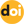The peer-reviewed scientific and technology journal. ISSN: 0204-3327

# On the Concept of «Effective Delayed Neutron Fraction»

6/20/2022 2022 - #02 Personnel traininghttps://doi.org/10.26583/npe.2022.2.16

### UDC: 621.039.514.4:621.039.515:621.039.516:378

The article considers methodological issues related to the conceptual and terminological apparatus of the dynamics of nuclear reactors. Based on an elementary analysis of the standard point reactor kinetics equations, the authors shows that it is necessary to clarify the physical meaning of the parameter β included in the equations, which is traditionally interpreted as the «effective delayed neutrons fraction» (EDNF). It follows directly from the kinetics equations that the parameter β, which appears in these equations as EDNF, is, from the point of view of the neutron balance, the fraction of prompt neutrons consumed for the generation of delayed neutron precursors (DNP), and, from the point of view of the DNP balance, the DNP yield per prompt neutron in a single fission event. With these interpretations taken into account, the role of the β parameter is considered in situations related with its adjustment by multiplying it by the «delayed neutron efficiency factor» and with the establishment of the actual fractions of prompt and delayed neutrons. In particular, it is shown that:

– the statement «if the delayed neutron fraction is β, then the prompt neutron fraction is equal to 1 – β» used in the problems of analyzing the nuclear reactor dynamics as a starting position, cannot be considered applicable to any reactor conditions;

– an increase in the β parameter by multiplying it by the «delayed neutron efficiency factor» leads, contrary to traditional interpretations, not to an increase but to a decrease in neutron reproduction in a supercritical reactor.

The proposed clarifications are appropriate both in terms of more adequate descriptions of processes in nuclear reactors, and in relation to the formulations of nuclear safety requirements.

1. Bahman Z. Neutronic Analysis For Nuclear Reactor Systems. Springer, 2019, 672 p.;DOI: https://doi.org/10.1007/978-3-030-04906-5.
2. Kerlin T.W., Upadhyaya B. R. Dynamics and Control of Nuclear Reactors. Academic Press, 2019, 402 p.; DOI: https://doi.org/10.1016/B978-0-12-815261-4.00011-1 .
3. Marguet S. The Physics of Nuclear Reactors. Springer Publ., 2017, 1462 p. DOI: https://doi.org/10.1007/978-3-319-59560-3 .
4. Seleznev E.F. Kinetics of Fast Neutron Reactors. Moscow. Nauka Publ., 2013, 239 p. (in Russian).
5. Popov A.K. Fundamentals of Nuclear Reactor Control. Moscow. MGU Publ., 2012, 208 p. (in Russian).
6. Sarkisov A.A., Puchkov V.N. Neutron-Physical Processes in Fast Reactors with Heavy Liquid-Metal Heat Carriers. Moscow. Nauka Publ., 2011, 168 p. (in Russian).
7. Vladimirov V.I. Physics of Nuclear Reactors: Practical Tasks for Their Operation. Moscow. LIBROCOM Publ., 2009, 480 p. (in Russian).
8. Halimonchuk V.А. Dynamics of Reactor with Distributed Parameters in Studies of Transient Operation Modes of VVER and RBMK. Kiev. Osnova Publ., 2008, 228 p. (in Russian).
9. Yurkevich G.P. Power Reactor Control Systems. Moscow. ELEKS-KM Publ., 2001, 344 p. (in Russian).
10. Merzlikin G.Ya. Fundamentals of the Theory of Nuclear Reactors. A Course for NPP Operating Personnel. Sevastopol. SIYaEiP Publ., 2001. – 343 pр. (in Russian).
11. Kolesov V.F., Leppik P.A., Pavlov S.P., Plyutinsky V.I., Sabaev E.F., Torlin B.Z., Shevelev Ya.V. Dynamics of Nuclear Reactors. Moscow. Energoatomizdat Publ., 1990, 518 pp. (in Russian).
12. Dementiev B. A. Kinetics and Regulation of Nuclear Reactors. Moscow. Energoatomizdat Publ., 1986, 272 p. (in Russian).
13. Sarkisov A.A., Puchkov V.N. Physics of Transients in Nuclear Reactors. Moscow. Energoatomizdat Publ., 1983, 264 p. (in Russian).
14. Bartolomey G.G., Bat G.A., Baibakov V.D., Alkhutov M.S. Fundamentals of Theory and Calculation Methods for Nuclear Power Reactors. Moscow. Energoizdat Publ., 1982, 512 p. (in Russian).
15. Emeliyanov I.Ya., Efanov A.I., Konstantinov L.V. Scientific and Technical Fundamentals of Nuclear Reactor Management. Moscow. Energoizdat Publ., 1981, 360 p. (in Russian).
16. Bell D., Glesson S. Theory of Nuclear Reactors. Moscow. Atomizdat Publ., 1974. 489 p. (in Russian).
17. Lewins J. Nuclear Reactor Kinetics and Control. Pergamon Press, 1978, 275 p.
18. Akcasu Z., Lellouche G.S., Shotkin L.M. Mathematical Methods in Nuclear Reactor Dynamics. Academic Press, 1971, 465 p.
19. Physics of Nuclear Reactors. Moscow. Atomizdat Publ., 1964, 384 p.
20. Glasston S., Edlund М. Foundations of the Theory of Nuclear Reactors. Moscow. Inostrannaya Literatura Publ., 1954, 458 p. (in Russian).
21. Toshinsky G.I., Bulavin P.E. Calculation of the Relative Importance of Delayed Neutrons in a Reactor. Atomic Energy.1967, v. 23, iss. 2, pp. 845-846; DOI: https://doi.org/10.1007/BF01179634 .
22. Yuferov A.G. About the Concept of «Prompt Criticality». Izvestiya vuzov. Yadernaya Energetika. 2021, no. 4, pp. 135-145; DOI: https://doi.org/10.26583/npe.2021.4.12(in Russian).

Link for citing the article: Yuferov A.G. On the Concept of «Effective Delayed Neutron Fraction». Izvestiya vuzov. Yadernaya Energetika. 2022, no. 2, pp. 174-182; DOI: https://doi.org/10.26583/npe.2022.2.16 (in Russian).